# sklearn.datasets.load_diabetes¶

sklearn.datasets.load_diabetes(return_X_y=False)[source]

Load and return the diabetes dataset (regression).

 Samples total 442 Dimensionality 10 Features real, -.2 < x < .2 Targets integer 25 - 346

Read more in the User Guide.

Parameters: return_X_y : boolean, default=False. If True, returns (data, target) instead of a Bunch object. See below for more information about the data and target object. New in version 0.18. data : Bunch Dictionary-like object, the interesting attributes are: ‘data’, the data to learn, ‘target’, the regression target for each sample, ‘data_filename’, the physical location of diabetes data csv dataset, and ‘target_filename’, the physical location of diabetes targets csv datataset (added in version 0.20). (data, target) : tuple if return_X_y is True New in version 0.18.

## Examples using sklearn.datasets.load_diabetes¶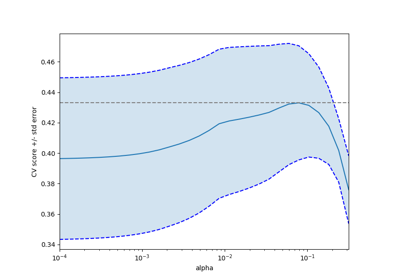Cross-validation on diabetes Dataset Exercise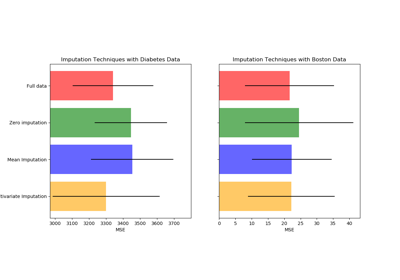Imputing missing values before building an estimator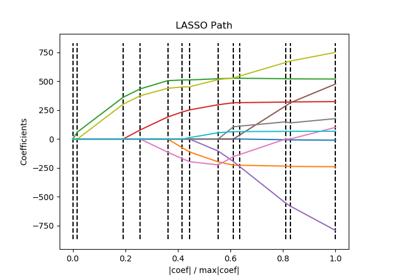Lasso path using LARS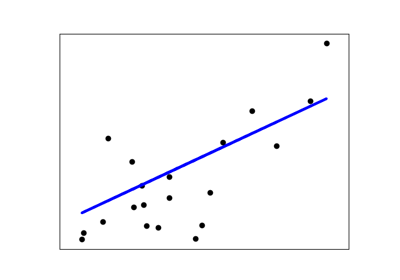Linear Regression Example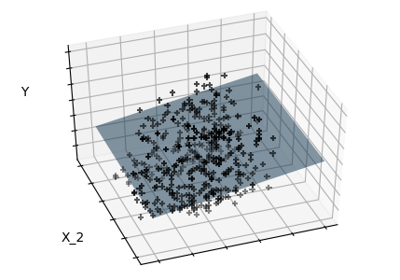Sparsity Example: Fitting only features 1 and 2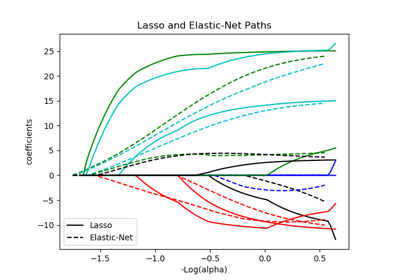Lasso and Elastic Net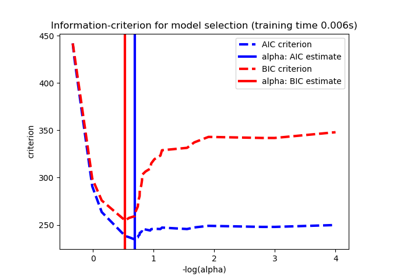Lasso model selection: Cross-Validation / AIC / BIC